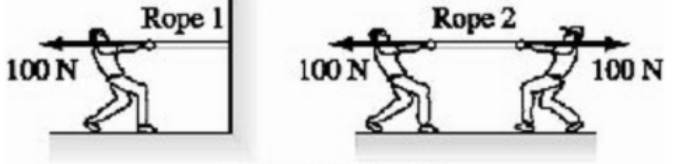# Problem: Question 1According to the discussion in our textbook, the general meaning of the term "acceleration constraint" is that, in some circumstances, we can say:a. two objects have to accelerate in the same direction.b. an object is prevented from accelerating.c. the acceleration of an object has to be positive.d. two objects must have accelerations of equal magnitude.  Question 2Consider the two ropes shown in the diagram. Is the tension in rope 2 greater than, less than, or equal to the tension in rope 1?a. Equal to.b. Greater than.c. Less than. Question 3The propulsion force on a car is due to:a. elastic energy.b. the car engine.c. kinetic friction.d. static friction.

###### FREE Expert Solution

Question 1

Acceleration constraint gives a well-defined relationship between the acceleration of two or more objects.

82% (64 ratings)###### Problem Details

Question 1

According to the discussion in our textbook, the general meaning of the term "acceleration constraint" is that, in some circumstances, we can say:

a. two objects have to accelerate in the same direction.

b. an object is prevented from accelerating.

c. the acceleration of an object has to be positive.

d. two objects must have accelerations of equal magnitude.

Question 2

Consider the two ropes shown in the diagram. Is the tension in rope 2 greater than, less than, or equal to the tension in rope 1?a. Equal to.

b. Greater than.

c. Less than.

Question 3

The propulsion force on a car is due to:

a. elastic energy.

b. the car engine.

c. kinetic friction.

d. static friction.